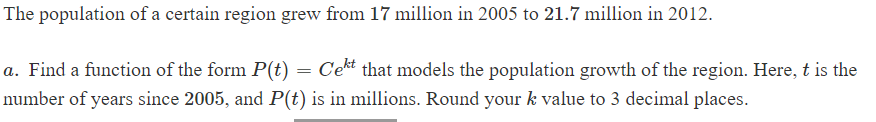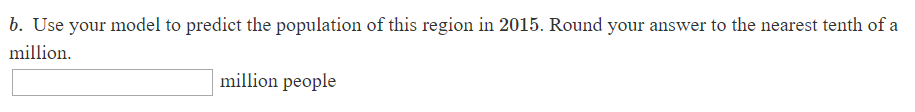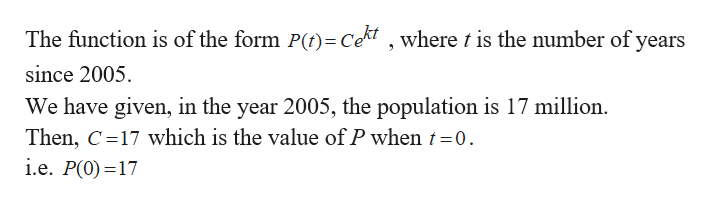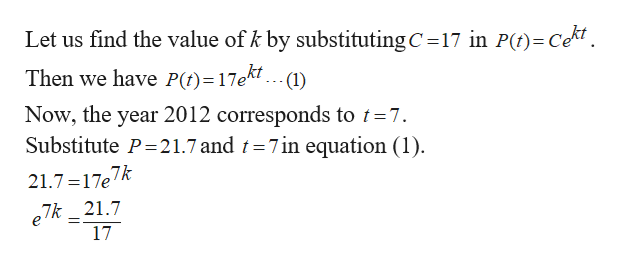# The population of a certain region grew from 17 million in 2005 to 21.7 million in 2012a. Find a function of the form P(t) = Cethat models the population growth of the region. Here, t is thenumber of years since 2005, and P(t) is in millions. Round your k value to 3 decimal places. b. Use your model to predict the population of this region in 2015. Round your answer to the nearest tenth of amillionmillion people

Question
27 viewshelp_outlineImage TranscriptioncloseThe population of a certain region grew from 17 million in 2005 to 21.7 million in 2012 a. Find a function of the form P(t) = Ce that models the population growth of the region. Here, t is the number of years since 2005, and P(t) is in millions. Round your k value to 3 decimal places. fullscreenhelp_outlineImage Transcriptioncloseb. Use your model to predict the population of this region in 2015. Round your answer to the nearest tenth of a million million people fullscreen
check_circle

Step 1
1. Given, the population P is 17 million in 2005 and has grown to 21.7 million in 2012, let us find the formula for P(t).
Step 2help_outlineImage TranscriptioncloseThe function is of the form P()Cekt , where t is the number of years since 2005 We have given, in the year 2005, the population is 17 million. Then, C 17 which is the value of P when t 0. i.e. P(0) 17 fullscreen
Step 3help_outlineImage TranscriptioncloseLet us find the value of k by substituting C 17 in Pt)=Cekt Then we have P(t)=17ekt ... (1) Now, the year 2012 corresponds to t =7. Substitute P 21.7 and t 7in equation (1) 21.7 17e7k 7k_21.7 17 =. fullscreen

### Want to see the full answer?

See Solution

#### Want to see this answer and more?

Solutions are written by subject experts who are available 24/7. Questions are typically answered within 1 hour.*

See Solution
*Response times may vary by subject and question.
Tagged in

### Applications of Mathematics